# Texas Go Math Kindergarten Unit 4 Assessment Answer Key

Refer to our Texas Go Math Kindergarten Answer Key Pdf to score good marks in the exams. Test yourself by practicing the problems from Texas Go Math Kindergarten Unit 4 Assessment Answer Key.

## Texas Go Math Kindergarten Unit 4 Assessment Answer Key

Vocabulary

Question 1.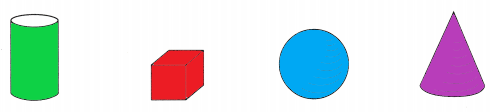Explanation:
Cylinder is colored in green
cube in red, sphere in blue and cone in purple.

Concepts and Skills

Question 2.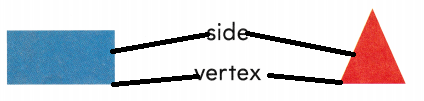Explanation:
Rectangle as 4 sides and 4 vertices
triangle has 3 sides and 3 vertices.

Question 3.Explanation:
Cube has flat surfaces
sphere has curved surfaces.

Question 4.Explanation:
A square has 4 sides and 4 vertices
all sides are equal in the square.

Directions
1. Use blue to color the sphere. Use green to color the cylinder. Use red to color the cube. Use purple to color the cone. TEKS K.6.B 2. Draw a line from the word side to a side of each shape. Draw a line from the word vertex
to a vertex, or corner, of each shape. TEKS K.6.D 3. Circle the words that describe a cube. TEKS K.6.B 4. I have four square vertices and four straight sides. What am I? Draw the shape. TEKS K6.D

Question 5.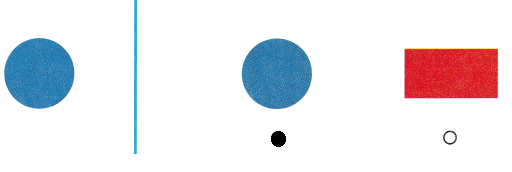Explanation:
The given shape is a circle
so, marked the circle.

Question 6.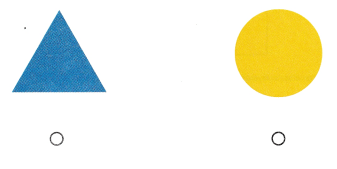Explanation:
A triangle has 3 sides and 3 vertices
so, marked the triangle.

Question 7.Explanation:
A cylinder has 2 flat surfaces and one curved surface.
A cone has one flat surface and a curved surface.

Question 8.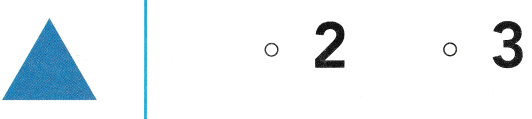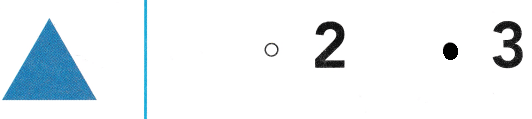Explanation:
A triangle has 3 sides and 3 vertices
so, marked the number 3.

Directions
5. Mark under the shape that matches the shape at the beginning of the row. TEKS K.6.A 6. Mark under the shape that is a triangle. TEKS K.6.A 7. Mark beside the number that shows how many flat surfaces the cylinder has. TEKS K.6.B 8. Mark beside the number that shows how many sides the triangle has. TEKS K.6.D

Texas Test Prep

Question 9.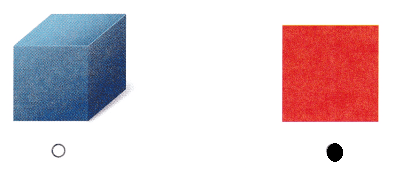Explanation:
A square has a flat surface
and a cube has 6 sides

Question 10.Explanation:
In first group there are cylinder and a cube
in the second group there is a sphere and a cube
so, marked the first cube.

Question 11.Explanation:
In a first group there is a cylinder and a cube
in the second group there is cube and a sphere
so, marked the first group.

Question 12.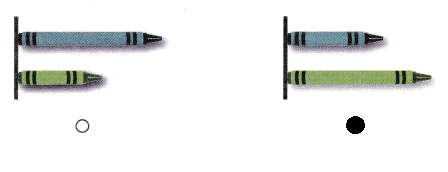Explanation:
In first group the blue crayon is longer than the green crayon
in second group the green crayon is longer than the blue crayon
so, marked the second group.

Directions
9. Mark under the shape that is flat. TEKS K.6.D 10. Mark under the set that shows a cube and a cylinder. TEKS K.6.B 11. Mark under the set that shows a cylinder and a cube. TEKS K.6.B 12. Mark under the set that shows the green crayon is longer than the blue crayon. TEKS K.7.B